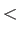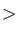### Keyword DOS

This keyword specifies the printing of the squares of the elements of the orthonormalized MO eigenvectors which are essential ingredients for generating a density of states.
Options: MOS=Integer1-Integer2A
 MOS=Integer1-Integer2Specification of orbital range for DOS calculation, first MO through last MO.
Description:
Use of the DOS keyword causes Löwdin-orthonormalized MO eigenvectors to be printed. As printed, each element is the square of the corresponding eigenvector component, i.e. the sum of the values of the printed eigenvector is unity. Thus, each element reflects the proportional contribution of an AO to the partial density represented by that one MO. A density of states (DOS) diagram can be created from these values. Printing of the vectors can be limited with the MOS option. The input MOS = <First> - <Last> specifies that only the MOs in the range <First> through <Last> are printed. Here <First> and <Last> refer to the (integer) indices of the molecular orbitals. For example, to print the vectors related to orbitals 10 through 25, the following input can be used:

``` DOS MOS=10-25
```